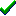# fg_3Dshade()Win32DirectXFastgraph/Light

Prototype

void fg_3Dshade (double *xyz_array, char *rgb_array, int n);

Sub fg_3Dshade (xyz_array() As Double, rgb_array() As Byte, ByVal n As Long)

procedure fg_3Dshade (var xyz_array : double; var rgb_array : byte; n : integer);

Description

The fg_3Dshade() function draws a Gouraud-shaded convex polygon defined in 3D world space, with optional z-buffering and 3D clipping. Backface removal is performed unless drawing a z-buffered polygon.

Parameters

xyz_array is an array containing the 3D world space (x,y,z) coordinates for each polygon vertex. The first three xyz_array elements represent the (x,y,z) values at the polygon's first vertex, the next three xyz_array elements are for the second vertex, and so forth.

rgb_array is an array containing the RGB color components for each polygon vertex. The first three rgb_array elements represent the RGB color values at the polygon's first vertex, the next three rgb_array elements are for the second vertex, and so forth. Each RGB color component is a value between 0 and 255.

n is the number of vertices in each of the above arrays.

Return value

none

Restrictions

If you attempt to fill a non-convex polygon with fg_3Dshade(), only a part of it will be filled.

See also

Examples

Contents
Fastgraph Home Page

copyright 2001 Ted Gruber Software, Inc.